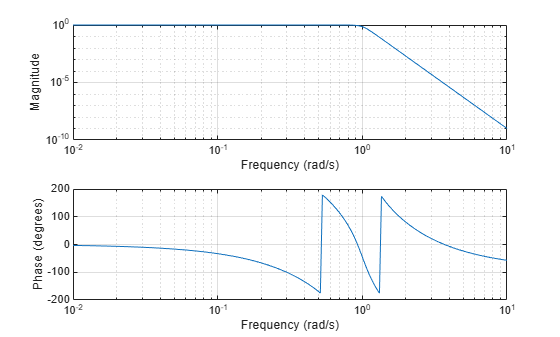# buttap

Butterworth filter prototype

## Syntax

``[z,p,k] = buttap(n)``

## Description

example

````[z,p,k] = buttap(n)` returns the poles and gain of an order `n` Butterworth analog lowpass filter prototype.```

## Examples

collapse all

Design a 9th-order Butterworth analog lowpass filter. Display its magnitude and phase responses.

```[z,p,k] = buttap(9); % Butterworth filter prototype [num,den] = zp2tf(z,p,k); % Convert to transfer function form freqs(num,den) % Frequency response of analog filter```## Input Arguments

collapse all

Order of Butterworth filter, specified as a positive integer scalar.

## Output Arguments

collapse all

Zeros of the system, returned as a matrix. `z` contains the numerator zeros in its columns. `z` is an empty matrix because there are no zeros.

Poles of the system, returned as a column vector. `p` contains the pole locations of the denominator coefficients of the transfer function.

Gains of the system, returned as a scalar. `k` contains the gains for each numerator transfer function.

## Algorithms

The function `buttap` returns the poles in the length `n` column vector `p` and the gain in scalar `k`. `z` is an empty matrix because there are no zeros. The transfer function is

`$H\left(s\right)=\frac{z\left(s\right)}{p\left(s\right)}=\frac{k}{\left(s-p\left(1\right)\right)\left(s-p\left(2\right)\right)\cdots \left(s-p\left(n\right)\right)}$`
```z = []; p = exp(sqrt(-1)*(pi*(1:2:2*n-1)/(2*n)+pi/2)).'; k = real(prod(-p)); ```

### Note

The function `buttap` returns zeros, poles, and gain ```(z, p, and k)``` in MATLAB®. However, the generated C/C++ code for `buttap` returns only poles `p` and gain `k` since zeros `z` is always an empty matrix.

Butterworth filters are characterized by a magnitude response that is maximally flat in the passband and monotonic overall. In the lowpass case, the first 2`n`-1 derivatives of the squared magnitude response are zero at ω = 0. The squared magnitude response function is

`${|H\left(\omega \right)|}^{2}=\frac{1}{1+{\left(\omega /{\omega }_{0}\right)}^{2n}}$`

corresponding to a transfer function with poles equally spaced around a circle in the left half plane. The magnitude response at the cutoff angular frequency ω0 is always $1/\sqrt{2}$ regardless of the filter order. `buttap` sets ω0 to 1 for a normalized result.

 Parks, T. W., and C. S. Burrus. Digital Filter Design. New York: John Wiley & Sons, 1987.